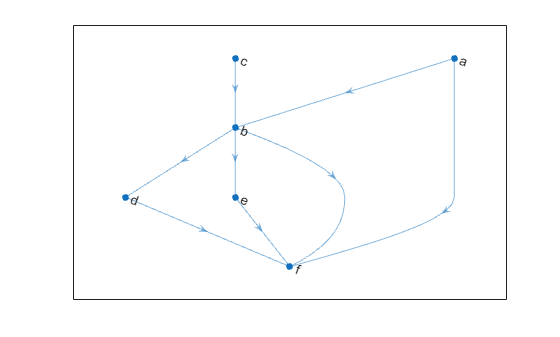# outdegree

Out-degree of nodes

## Syntax

``D = outdegree(G)``
``D = outdegree(G,nodeIDs)``

## Description

example

````D = outdegree(G)` returns a column vector containing the out-degree of each node in `G`.```

example

````D = outdegree(G,nodeIDs)` returns the out-degree of the nodes specified by `nodeIDs`.```

## Examples

collapse all

Create and plot a directed graph, and then compute the out-degree of every node in the graph. The out-degree of a node is equal to the number of edges with that node as the source.

```s = [1 3 2 2 4 5 1 2]; t = [2 2 4 5 6 6 6 6]; G = digraph(s,t); plot(G)````outdeg = outdegree(G)`
```outdeg = 6×1 2 3 1 1 1 0 ```

`outdeg(j)` indicates the out-degree of node `j`.

Create and plot a directed graph with named nodes. Then compute the number of edges that have the `'a'`, `'b'`, and `'f'` nodes as their source.

```s = {'a' 'c' 'b' 'b' 'd' 'e' 'a' 'b'}; t = {'b' 'b' 'd' 'e' 'f' 'f' 'f' 'f'}; G = digraph(s,t); plot(G)``````nodeID = {'a' 'b' 'f'}'; outdeg = outdegree(G,nodeID)```
```outdeg = 3×1 2 3 0 ```

`outdeg(j)` indicates the out-degree of node `nodeID(j)`.

## Input Arguments

collapse all

Input graph, specified as a `digraph` object. Use `digraph` to create a directed graph object.

Example: `G = digraph([1 2],[2 3])`

Node identifiers, specified as one or more node indices or node names.

This table shows the different ways to refer to one or more nodes either by their numeric node indices or by their node names.

FormSingle NodeMultiple Nodes
Node index

Scalar

Example: `1`

Vector

Example: `[1 2 3]`

Node name

Character vector

Example: `'A'`

Cell array of character vectors

Example: `{'A' 'B' 'C'}`

String scalar

Example: `"A"`

String array

Example: `["A" "B" "C"]`

Example: `outdegree(G,1)`

Example: `outdegree(G,["A" "B" "C"])`

## Output Arguments

collapse all

Out-degree of nodes, returned as a numeric array. `D` is a column vector unless you specify `nodeIDs`, in which case `D` has the same size as `nodeIDs`.